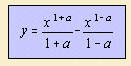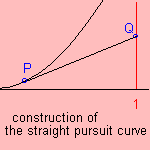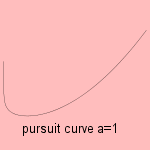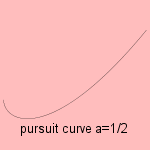# straight pursuit curve

## powerGiven a dog (P) chasing a pedestrian (Q). The anxious human creature runs in a straight line, with constant speed u. The dog runs also with a constant speed (v), always in the direction of its prey. The path the dog runs has been given the name pursuit curve. The ratio u/v of the two velocities is just the value of the parameter 'a' in the formula 1).
When the movement doesn't take place in a straight line, we can call the curve a generalized pursuit curve, to be distinguished from the straight pursuit curve on this page.

Leonardo da Vinci was the first to consider this problem.In some cases the curve degenerates to an algebraic curve, for instance when the parameter 'a' has the value 1/2.

Some authors confine the pursuit curve to the case that a = 1, when the velocities are the same. This leads to the following formula 2) :Arthur Bernhart studied the curve.

Realize that this pursuit curve (for a=1) is not the tractrix: equal distance between pursued and pursuer is not equivalent with equal speed between them.

notes

1)  the tangent to the curve of pursuit can be expressed as dy/dx = (y - ut) / (x -1)  (i)
The arc length of the curve of pursuit can be written as vt = √(1+(dy/dx)2))dx (ii)
Eliminate t form (i) and (ii), then set dy/dx = p(x) and differentiate (Kuipers 1966 p.360)

2) To understand that holds:realize thatwith f(x) = a x